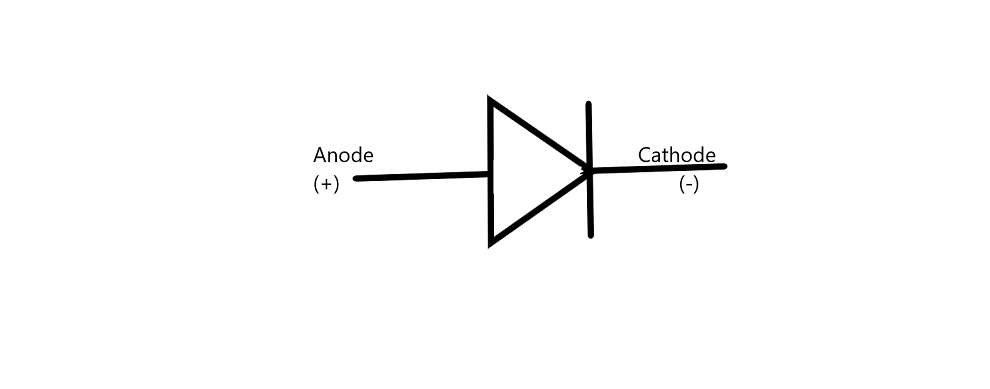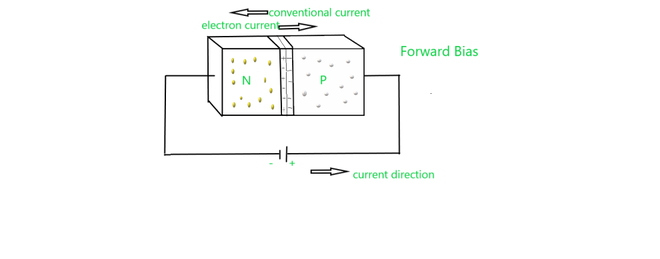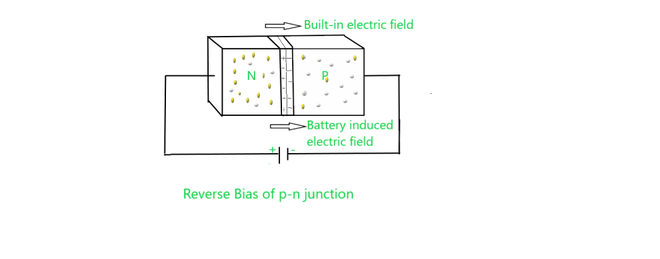What is a Diode? – Definition, Working, Types, Applications

• Last Updated : 07 Dec, 2021

Semiconductors have the resistivity lies between conductors and insulators. there is also the effect of temperature on the conductivity of semiconductor, when a suitable metallic impurity is added to it, The Conducting properties of Semiconductor changes. Semiconductors are of two types: Intrinsic semiconductors and Extrinsic semiconductors.

The outermost valence shell of an atom contain loosely bonded electron. when the valence electrons of such two types of atoms are bought close together, then the valence electrons of both these atoms combine to form “Electron pairs”. This type of bond is a covalent bond because they are weak in nature.

Some electrons tend to move out of their place and break the covalent bonds, due to the thermal energy supplied to the crystal. These broken covalent bonds, create free space, because of free electron which wanders randomly. this free space created by the removal of electrons is called a hole.

What is a Diode?

A diode is made up of two words i.e., “Di “ means Two, and “Ode “ means Electrodes which means that a device or component has two electrodes. (i.e., cathode and anode). A diode is an electronic device having a two-terminal unidirectional power supply. A semiconductor diode is the first diode that forms in semiconductor electronic devices, after that there were lots of new innovations takes place. but the most common diode used is a semiconductor diode.

A diode has two terminals that have a low resistance to the flow of the current in one direction, there is low resistance on one side and high resistance in the other, thus restricting the flow of current in one direction. Semiconductor diodes are two-terminal devices that consist of a p-n junction and metallic contacts at their two ends.

Materials that are used to make diode are: Germanium, silicon and germanium arsenide etc.

A p-n junction is known as a Semiconductor Diode. as it conducts only in one direction therefore it is used for the purpose of rectification. As it is made of a crystal-like Silicon or Germanium.  It is also known as a crystal diode. the symbol of the diode is as:Construction of Diode

We know that there are two types of semiconductor materials: Intrinsic and Extrinsic semiconductors. In intrinsic semiconductors number of electrons and holes concentration are equal at room temperature. In an extrinsic semiconductor, impurities are added to the semiconductor to increase the number of electrons or the number of holes. These impurities are pentavalent (Arsenic, Antimony, phosphorous) or tri-valent (boron, indium, aluminium).

A semiconductor diode has two layers. one layer is of p-type and the other is of n-type semiconductor.

• If we add trivalent impurities in a semiconductor (Silicon and germanium), a greater number of holes are present and it is a positive charge. therefore this type of layer is known as the p-type layer.
• If we add pentavalent impurities in semiconductors (silicon or germanium), due to excess electrons there is a negative charge. therefore this type of layer is known as the n-type layer.

Working of Diode

In the N-type region, the majority charge carriers are electrons and the minority charge carriers are holes. Whereas, In the P-type region, the majority of charge carriers are holes and negative charge carriers are electrons. Because of the concentration difference, the diffusion takes place in majority charge carriers and they recombine with the opposite charge. It makes a positive or negative ion. they collect near the junction. and this region is known as the depletion region.

• When anode or p-type of the diode is connected with a negative terminal and n-type or cathode is connected with the positive terminal of a battery, this type of diode is connected in reverse bias.
• when anode or p-type terminal is connected with a positive terminal and n-type or cathode is connected with the negative terminal of the battery, this type of diode is connected in forward bias.

Forward BiasForward bias

In biasing semiconductor is connected to external source. when the p-type semiconductor is connected to the positive terminal of the source or battery and negative terminal to the n-type, then this type of junction is said to be forward-biased. In forward bias the direction of built-in electric field near the junction and applied electric field are opposite in direction. this means that the resultant electric field has a magnitude lesser than the built-in electric field. due to this there is less resistivity and therefore depletion region is thinner. In silicon, at the voltage of 0.6 V, the resistance of the depletion region becomes completely negligible.

Reverse BiasReverse bias

In the reverse biasing, the n-type is connected to the positive terminal and the p-type is connected to the negative terminal of the battery . In this case, the applied electric field and the built-in electric field are in the same direction and the resultant of electric field has higher magnitude than the built-in electric field creating a more resistive, therefore depletion region is thicker. if the applied voltage becomes larger, then the depletion region becomes more resistive and thicker.

Unbiased diode

When there is no external source applied to semiconductors is known as an unbiased diode. the electric field is built up across the depletion layer between the p-type and the n-type material. this happens because of unbalanced no. of electrons and holes due to doping. At room temperature, for a silicon diode, 0.7V is barrier potential.

Types of Semiconductor Diode

There are different types of semiconductor diodes:-

1. LED – The term LED is Light Emitting Diode, it is the most useful kind of diode, when the diode is attached in forwarding bias, the then-current that flows through the junction produces light.
2. Zener diode – Zener diode is a type of diode, it allows the flow of current in forwarding direction, it can also work in the reverse breakdown but in breakdown condition, Zener diode has an application in voltage regulation, The Zener diode uses p-n junction in reverse bias mode, to give Zener effect.
3. Tunnel diode – The tunnel diode is used for microwave application.
4. Variable capacitance diode – This kind of diode are also called VARICAP diode, even though the output of variable capacitance can exhibit the general p-n junction diode but this diode is approved of giving the preferred capacitance change as they are different type of diode.
5. Photodiode – This kind of diode that produces current at a certain amount of light energy falls over it, In the condition of forwarding bias current transfer from p to n, while in reverse direction photocurrent flow in the reverse direction, there are two types of photodiode i.e., PN photodiode and PIN photodiode.
6. Switching diode etc.

Applications of Semiconductor Diode

Applications of semiconductor diode are as follow:

• Rectifier diode – Rectifier diode is a kind of diode that is used for the rectification of alternating current (A.C).
• LED – LED  is used for emitting an infrared light spectrum.
• Zener diode – Zener diode is used for the stabilization of current and voltage in electronic systems.
• Photodiode – works as a photodetector.
• Switching diode- which is used for fast switching requirements.
• A tunnel diode – A tunnel diode is a special type of diode which is used in the negative resistance region.

Sample Question

Question 1: Define the term Doping.

Doping is the process of adding impurities into the semiconductor, so that more electron-hole pair generate, The impurities added, are generally pentavalent and trivalent impurities. therefore they are p-type and n-type semiconductors.

Question 2: What happens when –

• The diode’s positive voltages are applied at the anode.
• The diode’s negative voltage is applied to the anode.

• This type of diode gets forward bias.
• This type of diode gets reverse bias.

Question 3: What is the effect of temperature on semiconductors:

• For Intrinsic semiconductor – the conductivity increases with the rise in temperature, because more number electron-hole pair generates.
• For Extrinsic semiconductors – When temperature increases the number of electron-hole pair increases which results in lesser effect of doping and more number of these pairs neutralizes.

Question 4: Define the term breakdown voltage of p-n junction.

In reverse bias condition, when the applied voltage increases gradually at a certain point there is increase in reverse current noticed, this is junction breakdown, corresponding applied voltage is known as breakdown voltage of p-n junction diode.

Question 5: What is the ratio of electrons and holes in the intrinsic semiconductor?